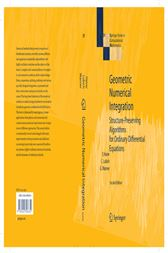Geometric Numerical Integration (2nd ed.)

Series: Springer Series in Computational Mathematics (No. 31)CA\$116.57
ISBNs
• 3540306668
• 9783540306634
• 9783540306665

Numerical methods that preserve properties of Hamiltonian systems, reversible systems, differential equations on manifolds and problems with highly oscillatory solutions are the subject of this book. A complete self-contained theory of symplectic and symmetric methods, which include Runge-Kutta, composition, splitting, multistep and various specially designed integrators, is presented and their construction and practical merits are discussed. The long-time behaviour of the numerical solutions is studied using a backward error analysis (modified equations) combined with KAM theory. The book is illustrated by many figures, it treats applications from physics and astronomy and contains many numerical experiments and comparisons of different approaches. The second edition is substantially revised and enlarged, with many improvements in the presentation and additions concerning in particular non-canonical Hamiltonian systems, highly oscillatory mechanical systems, and the dynamics of multistep methods.

ISBNs
• 3540306668
• 9783540306634
• 9783540306665

In The Press

From the reviews of the second edition:

"This book is highly recommended for advanced courses in numerical methods for ordinary differential equations as well as a reference for researchers/developers in the field of geometric integration, differential equations in general and related subjects. It is a must for academic and industrial libraries." -- MATHEMATICAL REVIEWS

"The second revised edition of the monograph is a fine work organized in fifteen chapters, updated and extended. … The material of the book is organized in sections which are … self-contained, so that one can dip into the book to learn a particular topic … . A person interested in geometrical numerical integration will find this book extremely useful." (Calin Ioan Gheorghiu, Zentralblatt MATH, Vol. 1094 (20), 2006)